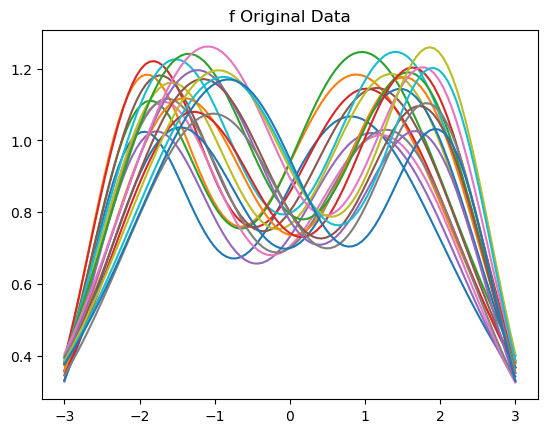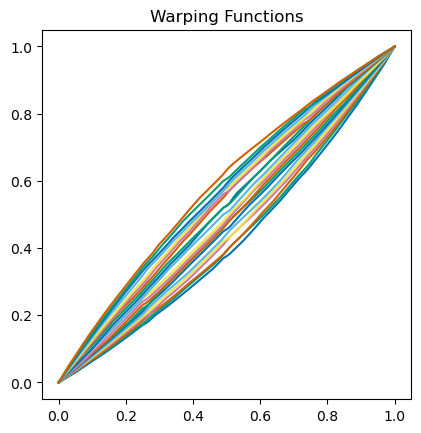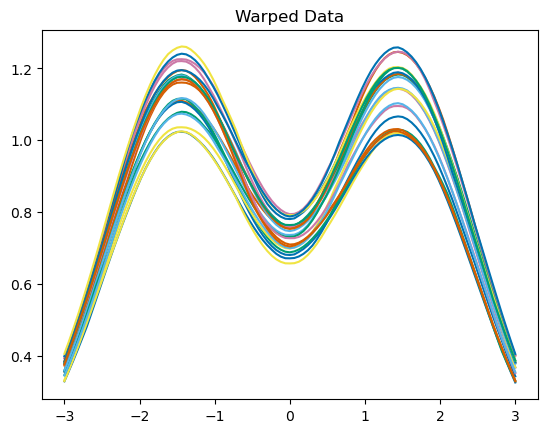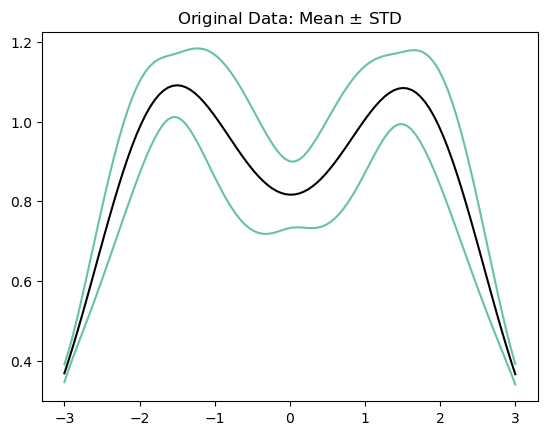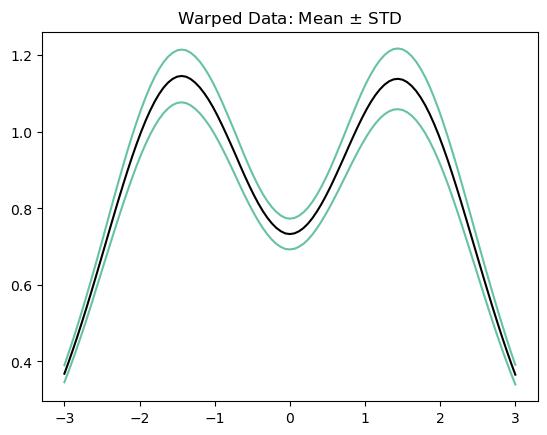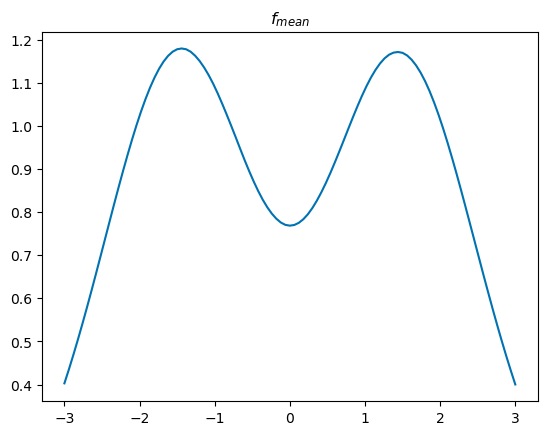# Elastic Functional Alignment¶

Otherwise known as time warping in the literature is at the center of elastic functional data analysis. Here our goal is to separate out the horizontal and vertical variability of the functional data

:

import fdasrsf as fs
import numpy as np


:

data = np.load('../../bin/simu_data.npz')
time = data['arr_1']
f = data['arr_0']


We will then construct the fdawarp object

:

obj = fs.fdawarp(f,time)


Next we will align the functions using the elastic framework

:

obj.srsf_align(parallel=True)

Initializing...
Compute Karcher Mean of 21 function in SRSF space...
updating step: r=1
updating step: r=2


Display plots demonstrating the alignment

:

obj.plot()